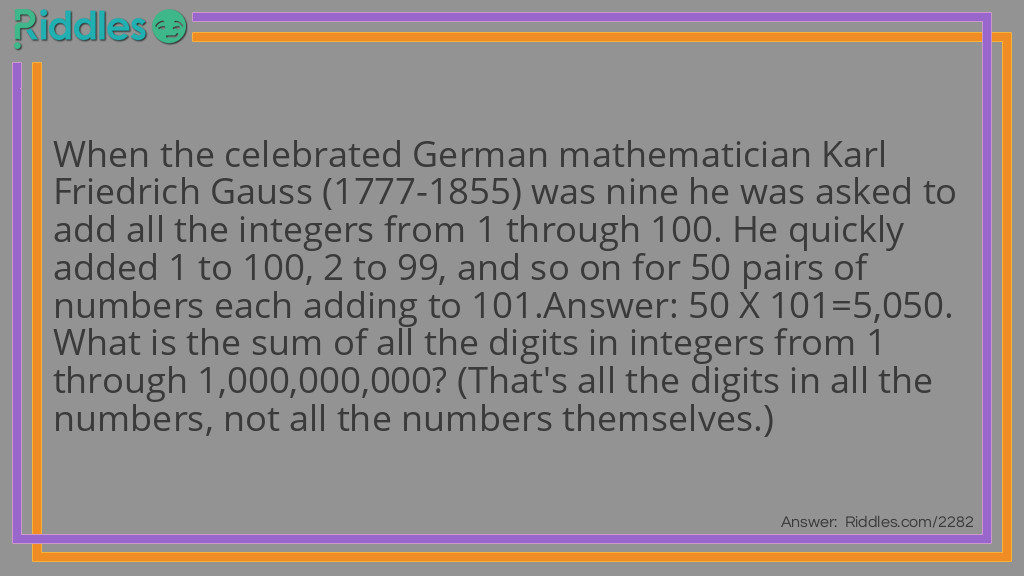# Mathematician Karl Friedrich Gauss Riddle 1 To 1,000,000,000

Author: Morgan Foster
7 years ago

Riddle: When the celebrated German mathematician Karl Friedrich Gauss (1777-1855) was nine he was asked to add all the integers from 1 through 100. He quickly added 1 to 100, 2 to 99, and so on for 50 pairs of numbers each adding to 101. Answer: 50 X 101=5,050. What is the sum of all the digits in integers from 1 through 1,000,000,000? (That's all the digits in all the numbers, not all the numbers themselves.)
Answer: The numbers can be grouped by pairs: 999,999,999 and 0; 999,999,998 and 1' 999,999,997 and 2; and so on.... There are half a billion pairs, and the sum of the digits in each pair is 81. The digits in the unpaired number, 1,000,000,000, add to 1. Then: (500,000,000 X 81) + 1= 40,500,000,001.
VOTE
Source: https://www.riddles.com/2282
COMMENTmathematician Karl Friedrich Gauss Riddle 1 To 1,000,000,000 by Morgan Foster v1.